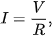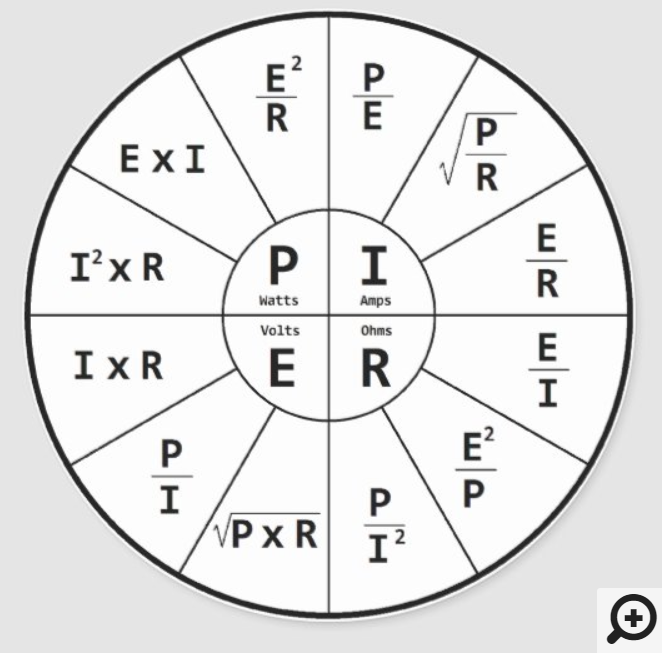Ohm's law states that the current through a conductor between two points is directly proportional to the voltage across the two points. Introducing the constant of proportionality, the resistance, one arrives at the usual mathematical equation that describes this relationship:

{\displaystyle I={\frac {V}{R}},}This chart shows the relevant equations used when calculating the size of a battery bank and the sufficient charge source(s):Watts is a function of Power.  There are three calculations for this:

I2 x R = P   (This is Current squared x Resistance = Power)
E x I = P (Volts x Current  = Power)
E2 / R = P (Volts squared / Resistance = Power)

EXAMPLE:

We know that Rolls 6-volt S6 L16-HC has a rated capacity (20 Hr AH rate or C/20) of 445 AH (current).

How many Watts of stored energy is this?

Volts x Current = Power

6V x 445 AH (C/20)  = 2,670 Watts of total stored energy.

A 24V series string of 6-volt S6 L16-HC batteries (4 x S6 L16-HC connected in series):

24V x 445 AH (C/20)  = 10,680 Watts of total stored energy.